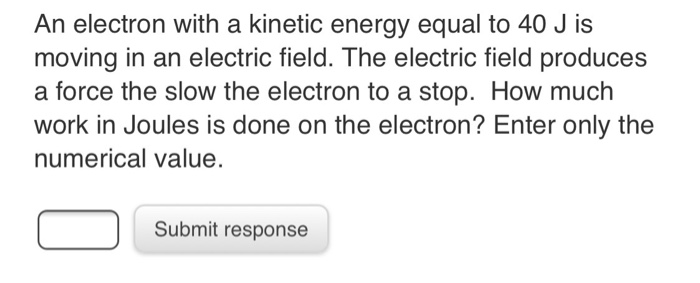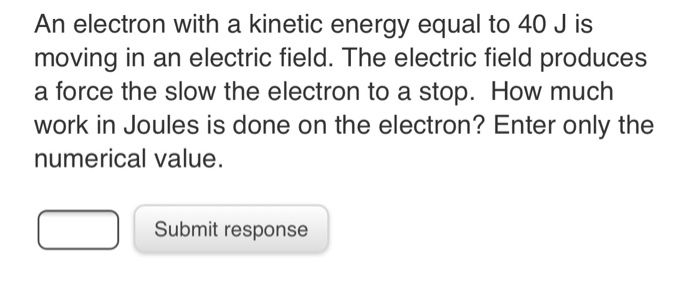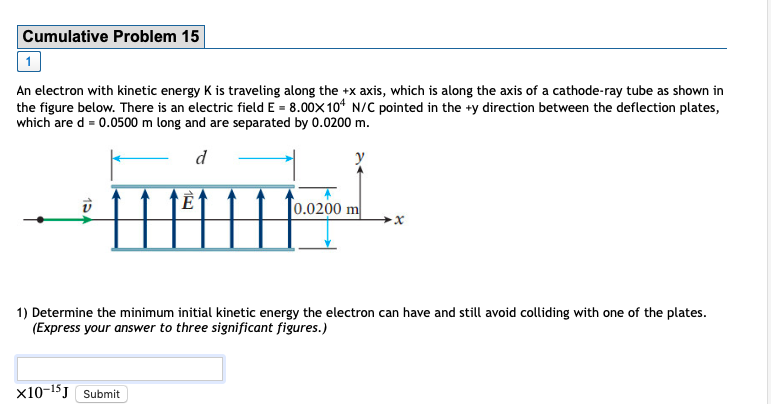Question

# Which field can change the kinetic energy of an electron? a. an electric field. b. a...

Which field can change the kinetic energy of an electron?
a. an electric field.
b. a magnetic field
c. both of them.
d. neither.
Which field can accelerate an electron, but not change its kinetic energy?
a. an electric field.
b. a magnetic field
c. both of them. d. neither.

1)

A) Electric field

2)

B) Magnetic field

#### Earn Coins

Coins can be redeemed for fabulous gifts.

Similar Homework Help Questions
• ### 1. An electron travels through an electric field. The kinetic energy of the electron changes from...

1. An electron travels through an electric field. The kinetic energy of the electron changes from 4.00 x 10-18 J to 3.95 x 10-18 J. Determine: a) the change in kinetic energy of the electron, b) the fall in electrical potential (voltage). c) its total energy (EPE + EC), at any point in its trajectory. (Remember the energy is conserved.) (EPEinitial = 0.00 J)

• ### An electron with a kinetic energy equal to 40 J is moving in an electric field....An electron with a kinetic energy equal to 40 J is moving in an electric field. The electric field produces a force the slow the electron to a stop. How much work in Joules is done on the electron? Enter only the numerical value. Submit response An electron with 50 J of energy enters a magnetic field. The magnetic field changes the direction of the electron to follow a helical path, but keeps a constant speed. How much work in...

• ### An electron with a kinetic energy equal to 40 J is moving in an electric field....An electron with a kinetic energy equal to 40 J is moving in an electric field. The electric field produces a force the slow the electron to a stop. How much work in Joules is done on the electron? Enter only the numerical value. Submit response An electron with 50 J of energy enters a magnetic field. The magnetic field changes the direction of the electron to follow a helical path, but keeps a constant speed. How much work in...

• ### Which statements are true for an electron moving in the direction of an electric field and...

Which statements are true for an electron moving in the direction of an electric field and why? (There may be more than one correct choice.) (a) Its electric potential energy increases as it goes from high to low potential. (b) Its electric potential energy decreases as it goes from high to low potential. (c) Its potential energy increases as its kinetic energy decreases. (d) Its kinetic energy decreases as it moves in the direction of the electric field. (e) Its...

• ### A proton and an electron are separately placed at rest in the same uniform electric field....

A proton and an electron are separately placed at rest in the same uniform electric field. What can be said about the energy of each after they are released? A) Electric potential energy of both decreases, and kinetic energy of both increases. B) Electric potential energy of both increases and kinetic energy of both decreases. C) Electric potential energy and kinetic energy of both remain constant. D) Electron potential energy increases, proton potential energy decreases. E) Electron potential energy decreases,...

• ### An electron with kinetic energy 228 eV is moving in a horizontal direction. The electron moves...

An electron with kinetic energy 228 eV is moving in a horizontal direction. The electron moves into a region where there is a uniform vertical electric field which has magnitude 3990 N/C. Find the smallest magnitude of a magnetic field that will cause the electron to continue to move horizontally. (1eV=1.6^10^(-19)J;mass of the electron=9.11*10^(-31)kg)

• ### An electron acquires 6.90×10-16 J of kinetic energy when it is accelerated by an electric field...

An electron acquires 6.90×10-16 J of kinetic energy when it is accelerated by an electric field from plate A to plate B. What is the potential difference between the plates? = _________________ V Which plate is at the higher potential?

• ### An electron with kinetic energy K is traveling along the +x axis, which is along the...An electron with kinetic energy K is traveling along the +x axis, which is along the axis of a cathode-ray tube as shown in the figure below. There is an electric field E = 8.00××1044 N/C pointed in the +y direction between the deflection plates, which are d = 0.0500 m long and are separated by 0.0200 m. Cumulative Problem 15 An electron with kinetic energy K is traveling along the +x axis, which is along the axis of a...

• ### An electron acquires 7.90×10−16 J of kinetic energy when it is accelerated by an electric field...

An electron acquires 7.90×10−16 J of kinetic energy when it is accelerated by an electric field from plate A to plate B.

• ### When electron moves in the direction which is against direction of electric field lines we can...

When electron moves in the direction which is against direction of electric field lines we can conclude that: c. it moves from high potential to low potential and its electric energy increases. it moves from high potential to low potential and its electric energy decreases. e. both its electric potential and electric potential energy stay constant. it moves from low potential to high potential and its electric energy increases. it moves from low potential to high potential and its electric...